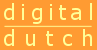# Bookmark Collection

## Math for Elementary Education

Internet resources for Elementary Mathematics
Share

### Collection Content

•Rubber Sheet Geometry (Presentation)
Intro to topology concepts
•Topology for Tots (Presentation)
Intro to topology concepts
•Maths aMazes (Presentation)
Mazes and Nets
•AlexWarp Code (Simulation)
Image distorrion w/source code
•WWW Unit Converter (Reference Material)
Unit conversions
•A Dictionary of Units (Reference Material)
unit Conversions
•Interactive Resources (Animation)
Nice activites with interactive ruler, protractor, and compass.
•The Vernier Caliper (Simulation)
Interactive Vernier Caliper
•Vernier Calipers (Simulation)
Interactive Vernier caliper
•Micrometer Calipers (Simulation)
Interactive Micrometer calipers
•Interactive Math Dictionary using Flash
•Cut-the-Knot! (Collection)
•Puzzle Pages (Collection)
Good pentomino and tangram info.
••Origami Cube (Simulation)
Directions for folding a cube.
•Interactive Java Applets
•See NCTM Standards.
•Pattern Blocks (Simulation)
•Interactive polyhedra
•Isometric Drawing Tool (Simulation)
Block and Mat diagrams.
•Geodesic Clubhouse (Simulation)
Construct a more complex geodesic dome from newsprint.
•Geodesic Dome (Simulation)
Construct a simple geodesic dome from newsprint.
••Virtual Manipulatives (Simulation)
This is a virtual manipulatives library. It contains virtual manipulatives for Numbers/Operations, Algebra, Geometry,... More
•Conceptua Math (Animation)
This is a group of fraction tools. Different tools address finding common denominators, adding fractions, subtracting... More